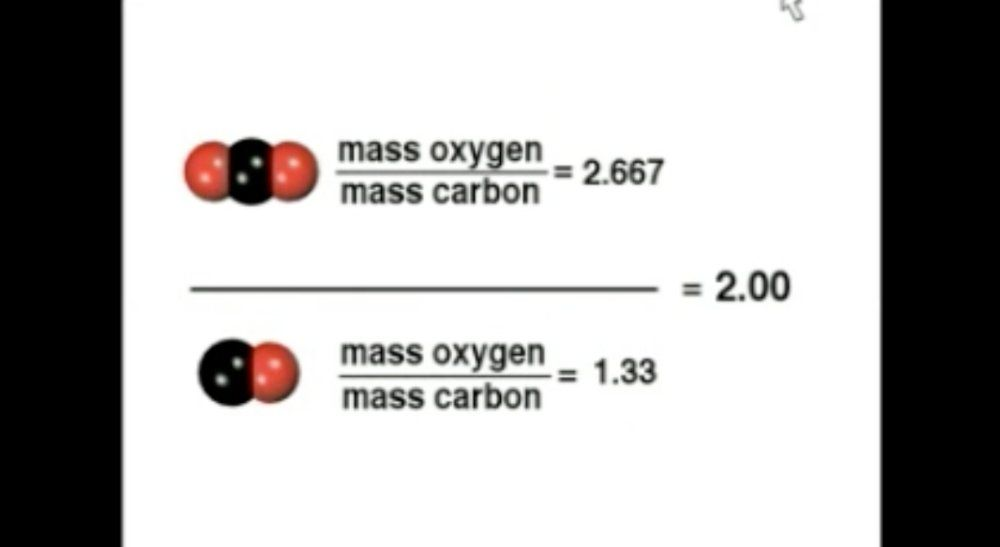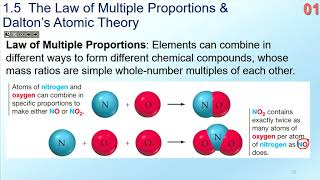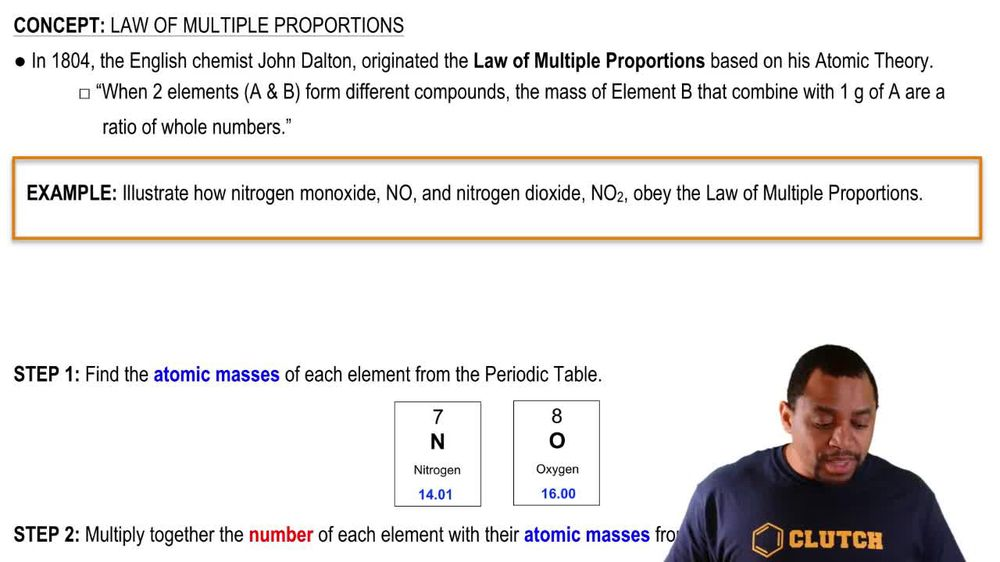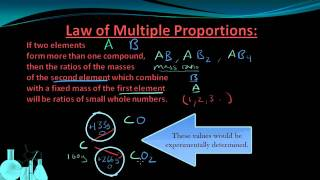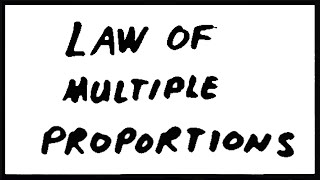Start typing, then use the up and down arrows to select an option from the list.
1. 2. Atoms & Elements2. Law of Multiple Proportions
Problem

# A compound containing sulfur and fluorine contains 8.00 g of S and 9.50 g of F. Which combination of S and F masses represents a different compound that obeys the Law of Mul-tiple Proportions? (LO 2.8) (a) 32.0 g of S and 38.0 g of F (b) 4.00 g of S and 4.75 g of F (c) 8.00 g of S and 10.5 g of F (d) 16.0 g of S and 57.0 g of F

Relevant Solution2m
Play a video:
Hi everyone for this problem we're told that silicon sulfide has a ratio of sulfur to silicon by mass equal 1.14-1. And an unknown has a ratio of sulfur to silicon by mass equal 3.5-1. What is its formula? So here we're dealing with what's called the law of multiple proportions. And what this law tells us is that when two elements combined with each other to form two or more compounds, the ratio is a simple whole number. And this ratio is what's used to inform us of what is our formula. So in this case we have our two elements silicon and sulfide and our two compounds is unknown and the unknown. So if we take the ratio between our two compounds, we'll get a simple whole number. Here's what I mean. So we have our unknown and are known, We're told that are unknown. Has a ratio of sulfur to silicon by mass equal 3.5-1. So our unknown is 3.5 sulfur to silicon. And are known is 1. sulfur to silicon. So once we do that we get a simple whole number of three. And this three is our sulfur. Because the problem tells us that our ratio of sulfur to silicon. Okay, and so now we need to figure out what is our formula. And this simple whole number that we just calculated is our number of sulfur to silicon. So we have three sull furs per one Silicon. So if we write that out as a formula we have for every one silicon, we have three cell furs. So that leaves us with a formula of S. I. S. Three. And this is our final formula, based off the ratio that we calculated. Okay, that's the end of this problem. I hope this was helpful.